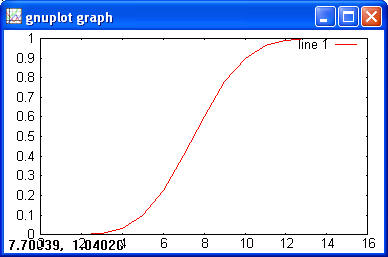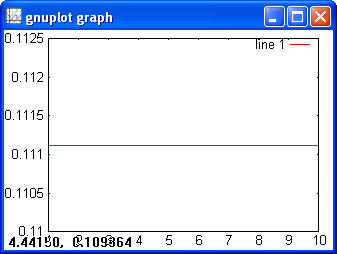numEclipse - An Eclipse based workbench for

Numerical Computing

# Statistics

This chapter introduces the statistics toolbox. This toolbox is based on Jakarta Commons Math project.

## 9.1 Probability Distributions

There are two type distributions, continuous and discrete. The statistics toolbox supports following distributions.

 Discrete Distributions Continuous Distributions Binomial Beta Geometric Cauchy Hypergeometric Chi-Square Poisson Exponential F Gamma Normal T Uniform Weibull

This toolbox provides following four functions for each of the distribution.

• Probability density function (pdf)

• Cumulative distribution function (cdf)

• Inverse cumulative distribution function (inv)

• Random number generator (rnd)

### 9.1.1  Discrete Distributions

#### Binomial

> x = 1:100;

> y = binopdf(x, 100, 0.5);

> plot(x, y)> y = binocdf(x, 100, 0.5)

> plot(x, y)> x = 0:0.01:0.9;

> y = binoinv(x, 100, 0.5)

> plot(x, y)

> x = 1:5;

> y = binocdf(x, 10, 0.5);

> binoinv(y, 10, 0.5)

> ans =

1   2   3   4   5

> y = binornd(100, 0.5, 1, 10)

> y =

51   53   59   43   49   49   52   45   63   47

#### Geometric

> x = 1:10;

> y = geopdf(x, 0.5);

> plot(x, y);> y = geocdf(x, 0.5)

> plot(x, y)> y = geocdf(1:10, 0.5)

> x = geoinv(y, 0.5)

> x =

1   2   3   4   5   6   7   8   9   10

> geornd(0.05, 1, 5)

> ans =

2   16   21   0   190

#### Hypergeometric

> x = 0:15;

> y = hygepdf(x, 100, 40, 20);

> plot(x, y)> y = hygecdf(x, 100, 40, 20)

> plot (x, y)> x = 1:5;

> y = hygecdf(x, 100, 20, 10);

> hygeinv(y, 100, 20, 10)

> ans =

1   2   3   4   5

> hygernd(100,40,50)

> ans =

18

#### Poisson

> x = 1:40;

> y = poisspdf(x, 10);

> plot(x, y)> y = poisscdf(x, 10)

> plot(x, y)> x = 1:10;

> y = poisscdf(x, 3);

> poissinv(y, 3)

> ans =

1   2   3   4   5   6   7   8   9   10

> poissrnd(3, 1, 5)

> ans =

6   0   3   1   4

### 9.1.2 Continuous Distributions

#### Beta

> a = 2;

> b = 5;

> x = 0:0.01:1;

> y = betapdf(x, a, b);

> plot(x, y)Other functions are not implemented at this point.

#### Chi-square

> x = 0:0.1:5;

> y = chi2pdf(x, 1);

> plot(x, y)> y = chi2cdf(x, 1);

> plot(x, y)> chi2cdf(1, 1)

> ans =

0.6827

> chi2inv(0.6827, 1)

> ans =

1.0000

> chi2rnd(1, [2 3])

> ans =

0.0207 5.2950 2.2307

0.0382 0.2803 0.0084

#### Exponential

> x = 0:0.1:10;

> y = exppdf(x, 3);

> plot(x, y)> y = expcdf(x, 3);

> plot (x, y)> x = 1:5;

> y = expcdf(x, 2);

> expinv(y, 2)

> ans =

1   2   3   4   5

#### F

> x = 0:0.1:100;

> y = fpdf(x, 2, 1);

> plot(x, y)> y = fcdf(x, 2, 1)> y = fcdf(1, 1, 10)

> ans =

0.6591

>finv(0.6591, 1, 10)

> ans =

1.0000

#### Gamma

> x = 0:0.1:20;

> y = gampdf(x, 2, 2);

> plot(x, y)> y = gamcdf(x, 2, 2)

> plot(x, y)> gamcdf(1, 2, 2)

> ans =

0.0902

> gaminv(0.0902, 2, 2)

> ans =

1.0000

#### Normal

> x = -5:0.1:5;

> y = normpdf(x);

> plot(x, y)> y = normcdf(x)

> plot(x, y)> normcdf(1)

> ans =

0.8413

> norminv(0.8413)

> ans =

0.9998

#### T

> x = -5:0.1:5;

> y = tpdf(x, 2);

> plot(x, y)> y = tcdf(x, 2);

> plot(x, y)> tcdf(1, 2)

> ans =

0.7887

> tinv(0.7887, 2)

> ans =

1.0001

#### Uniform

> x = 1:10;

> y = unifpdf(x, 1, 10);

> plot(x, y)> y = unifcdf(x, 1, 10);

> plot(x, y)> unifcdf(2, 1, 10)

> ans =

0.1111

> unifinv(0.1111, 1, 10)

> ans =

1.9999

#### Weibull

> x = 0:0.1:5;

> y = weibpdf(x, 0.1, 3);

> plot(x, y)> y = weibcdf(x, 0.1, 3);

> plot(x, y)> weibcdf(1, 1, 3)

> ans =

0.6321

> weibinv(0.6321, 1, 3)

> ans =

1.0000

## 9.2 Descriptive Statistics

numEclipse implements the following few functions for Descriptive statistics.

#### Mean

This method calculates the average value(s) for a matrix. The syntax is as follows

m = mean(X), or

m = mean(X, dim)

If X is a vector then "mean(X)" returns the mean value of X. In case of a matrix, it will return a row vector containing mean values of a corresponding columns in X.

"mean(X, dim)" allows to calculate mean along different dimensions of the matrix. For dim = 1, mean will be calculated along columns of X. For dim = 2, mean will be calculated along rows of X and the mean (X, dim) will return a column vector.

Example:

> x = [ 8 6 4; 0 4 9; 6 1 9];

> mean(x)

ans =

4.6667   3.6667   7.3333

> mean(x, 2)

ans =

6

4.3333

5.3333

#### Median

This method calculates the median value(s) for a matrix. The syntax is as follows

m = median(X), or

m = median(X, dim)

If X is a vector then "median(X)" returns the median value of X. In case of a matrix, it will return a row vector containing median values of a corresponding columns in X.

"median(X, dim)" allows to calculate median along different dimensions of the matrix. For dim = 1, median will be calculated along columns of X. For dim = 2, median will be calculated along rows of X and the median (X, dim) will return a column vector.

Example:

> x = [ 8 6 4; 0 4 9; 6 1 9];

> median(x)

ans =

6   4   9

> median(x, 2)

ans =

6

4

6

#### Var

This method calculates the variance value(s) for a matrix. The syntax is as follows

m = var(X), or

m = var(X, 1)

If X is a vector then "var(X)" returns the variance of X. In case of a matrix, it will return a row vector containing variance values of a corresponding columns in X.

"var" method normalizes by n-1 where n is the number of data values. Using "var(X, 1)", we can normalize by n.

Example:

> x = [ 8 6 4; 0 4 9; 6 1 9];

> var(x)

ans =

17.3333   6.3333   8.3333

> var(x, 1)

ans =

11.5556   4.2222   5.5556

#### std

This method calculates the standard deviation value(s) for a matrix. The syntax is as follows

m = std(X), or

m = std(X, 1)

If X is a vector then "std(X)" returns the standard deviation of X. In case of a matrix, it will return a row vector containing standard deviation values of a corresponding columns in X.

"std" method normalizes by n-1 where n is the number of data values. Using "std(X, 1)", we can normalize by n.

Example:

> x = [ 8 6 4; 0 4 9; 6 1 9];

> std(x)

ans =

4.1633   2.5166   2.8868

> std(x, 1)

ans =

3.3993   2.0548   2.3570

#### cov

This method calculates the covariance value(s) for a matrix. The syntax is as follows

m = cov(X), or

m = cov(X, Y)

"cov(X)" calculates the covariance matrix for X, where each column is considered as an observed values of a variable.

"cov(X,Y)" calculates the column-wise covariance between X and Y.

Example:

> x = [ 8 6 4; 0 4 9; 6 1 9];

> cov(x)

ans =

-2.0000   -4.0000   -5.6667

-5.0000   -1.0000    2.1667

5.0000    2.5000     -4.1667

## 9.3 Statistical Tests

numEclipse implements only two methods for statistical tests, i.e., t-test and z-test. These methods are adopted from octave.

### t-test

Octave help provides following text.

Function File: [PVAL, T, DF] = ttest (X, M, ALT)
For a sample X from a normal distribution with unknown mean and
variance, perform a t-test of the null hypothesis `mean (X) == M'.
Under the null, the test statistic T follows a Student
distribution with `DF = length (X) - 1' degrees of freedom.

With the optional argument string ALT, the alternative of interest
can be selected. If ALT is `"!="' or `"<>"', the null is tested
against the two-sided alternative `mean (X) != M'. If ALT is
`">"', the one-sided alternative `mean (X) > M' is considered.
Similarly for "<", the one-sided alternative `mean (X) < M' is
considered, The default is the two-sided case.

The p-value of the test is returned in PVAL.

### z-test

Octave help provides following text.

Function File: [PVAL, Z] = ztest (X, M, V, ALT)
Perform a Z-test of the null hypothesis `mean (X) == M' for a
sample X from a normal distribution with unknown mean and known
variance V. Under the null, the test statistic Z follows a
standard normal distribution.

With the optional argument string ALT, the alternative of interest
can be selected. If ALT is `"!="' or `"<>"', the null is tested
against the two-sided alternative `mean (X) != M'. If ALT is
`">"', the one-sided alternative `mean (X) > M' is considered.
Similarly for `"<"', the one-sided alternative `mean (X) < M' is
considered. The default is the two-sided case.

The p-value of the test is returned in PVAL.